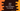# Java program to find out the top 3 numbers in an array## Java program to find out the top 3 numbers in an array :

In this tutorial, we will learn how to find out the top 3 numbers in an array. Algorithm used in this program is as below :

### Algorithm to find out the top 3 numbers :

1. In this program we are using method ‘findTopThree(int[] arr)’ to find out the top 3 elements of the array ‘arr’

2. Scan the elements one by one

3. Create three numbers ‘first’, ‘second’ and ‘third’ to store the first, second and third biggest element .

4. If any number is bigger than ‘first’, move second number to third, first number to second and set this number as first.

5. If any number is smaller than first but bigger than second, move second number to third and set this value as second.

6. If any number is smaller than first and second but bigger than third, set it as third.

7. After all numbers are scanned, print first ,second and third numbers.

### Sample Program to find out top 3 numbers:

``````import java.util.Scanner;

public class Main {

/**
* Utility functions for System.out.println() and System.out.print()
*/
private static void print(String str) {
System.out.print(str);
}

private static void println(String str) {
System.out.println(str);
}

private static void findTopThree(int[] arr) {
int first = 0;
int second = 0;
int third = 0;

for (int i = 0; i < arr.length; i++) { if (arr[i] > first) {
third = second;
second = first;
first = arr[i];
} else if (arr[i] > second) {
third = second;
second = arr[i];
} else if (arr[i] > third) {
third = arr[i];
}
}

println("First : " + first);
println("Second : " + second);
println("Third : " + third);
}

public static void main(String args[]) {
int total = 0;
Scanner sc = new Scanner(System.in);
println("How many numbers you want to add ? ");

total = sc.nextInt();

int[] arr = new int[total];

for (int i = 0; i < total; i++) {
println("Enter no " + (i + 1) + " : ");
arr[i] = sc.nextInt();
}

println("Top three numbers for the array : ");
findTopThree(arr);
}
}
``````

### Output :

``````How many numbers you want to add ?
9
Enter no 1 :
8
Enter no 2 :
6
Enter no 3 :
7
Enter no 4 :
4
Enter no 5 :
5
Enter no 6 :
3
Enter no 7 :
2
Enter no 8 :
1
Enter no 9 :
10
Top three numbers for the array :
First : 10
Second : 8
Third : 7
``````